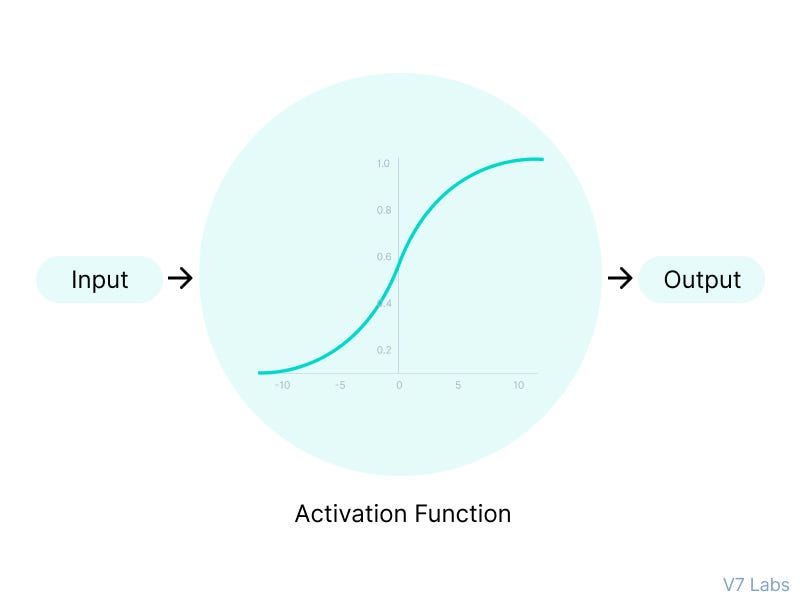# Unfolding Neural Networks: A Comprehensive Guide to Understanding Activation Functions### Introduction

At the heart of a neural network, the mechanism that allows it to learn complex patterns, solve intricate problems, and make predictions is a set of mathematical operations called activation functions. An activation function in a neural network decides whether a neuron should be activated or not by calculating the weighted sum and further adding bias with it. The purpose of the activation function is to introduce non-linearity into the output of a neuron, which is essential because most real-world data is non-linear. This comprehensive guide will provide an in-depth look at the role, types, and implications of activation functions in neural networks.

### Understanding Activation Functions

An activation function is a fundamental component of a neural network, determining the output of a neuron, or “node,” based on its input. Essentially, it transforms the inputs of the node, which are the outputs of the previous layer, into an output that is passed onto the next layer.

The primary purpose of an activation function is to add a degree of complexity to the network, allowing it to learn from the error that it makes and adjust its weights accordingly. Without an activation function, a neural network would merely be a linear regression model, unable to handle complex tasks.

### Linear and Non-Linear Activation Functions

Activation functions can be broadly classified as linear or non-linear. A linear activation function takes the form of a straight line, meaning it can only solve problems where the output is a linear combination of the inputs. However, most real-world problems are non-linear, making linear activation functions relatively limited in their use.

Non-linear activation functions, on the other hand, allow neural networks to learn from errors and make necessary adjustments. They enable the model to handle more complex tasks, such as image and speech recognition or natural language processing, and can be further divided into several types:

#### 1. Sigmoid Activation Function

The sigmoid activation function is one of the most widely used non-linear activation functions. It maps the input values to outputs that range between 0 and 1, giving an S-shaped curve. This characteristic allows the network to predict probabilities, making it particularly useful in binary classification problems.

#### 2. Tanh Activation Function

Tanh, or hyperbolic tangent, is similar to the sigmoid function but maps the input values to outputs between -1 and 1. This function provides better outputs for negative input values compared to the sigmoid function.

#### 3. ReLU Activation Function

ReLU, or Rectified Linear Unit, is one of the most commonly used activation functions in convolutional neural networks (CNNs) and deep learning. ReLU is computationally efficient and helps to mitigate the vanishing gradient problem, a common issue where the lower layers of a network train very slowly because the gradient decreases exponentially through the layers.

#### 4. Leaky ReLU Activation Function

Leaky ReLU is a variant of ReLU that solves the “dying ReLU” problem, where a large gradient update can cause a neuron to become stuck perpetually on zero output. Instead of defining the function as zero for negative inputs, Leaky ReLU allows a small, non-zero output.

#### 5. Softmax Activation Function

The softmax activation function is particularly useful in the output layer of a network for multi-class classification problems. It converts the outputs of each unit to range between 0 and 1, just like a sigmoid function. But it also divides each output by the sum of the outputs, which gives the probability distribution over multiple classes.

### Choosing an Activation Function

The choice of activation function depends on the specific requirements of the neural network and the type of problem it’s being used to solve. For example, ReLU is a popular choice for many scenarios due to its simplicity and performance across a wide range of tasks. However, it’s not always suitable. For example, in cases where negative input values may be significant, a leaky ReLU or tanh function might be more appropriate. For output layers, the softmax function is often used for multi-classification problems, while the sigmoid function can be used for binary classification problems.

### Conclusion

In the realm of neural networks, activation functions play a vital role in adding complexity to the model, enabling it to learn and adapt from its errors. Understanding different types of activation functions, their benefits, and their use-cases is essential for anyone working with neural networks. As a practitioner, being able to select the right activation function for a particular neural network can make a significant difference in the network’s performance, leading to more accurate and reliable predictions.

# Personal Career & Learning Guide for Data Analyst, Data Engineer and Data Scientist

## Applied Machine Learning & Data Science Projects and Coding Recipes for Beginners

A list of FREE programming examples together with eTutorials & eBooks @ SETScholars

# Projects and Coding Recipes, eTutorials and eBooks: The best All-in-One resources for Data Analyst, Data Scientist, Machine Learning Engineer and Software Developer

Topics included:Classification, Clustering, Regression, Forecasting, Algorithms, Data Structures, Data Analytics & Data Science, Deep Learning, Machine Learning, Programming Languages and Software Tools & Packages.
(Discount is valid for limited time only)## Find more … …

SQL tutorials for Business Analyst – SQL | Introduction

Non-Linear Regression in R – random forest algorithm in R

Non-Linear Regression in R – gradient boosted machine in R